# MySQL SUM()

The `SUM()` aggregate function returns the sum of an expression.

If the return set has no rows, the `SUM()` function returns `NULL`.

Consider the following result set: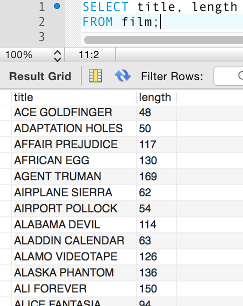You can see that the `film` table contains a column for storing the length (in minutes) of each film.

We could use the `SUM()` function to return the total length of all films combined.

Result: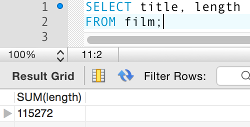You could even divide that number by 60 to give you the number of hours (we also round it with `ROUND()`):

Result: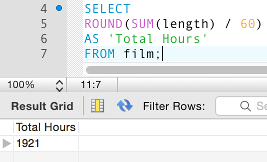Maybe the movie rental provider could use this info to boast that they have "Over 1920 hours worth of movies!".

## Another Example

Consider the following result set: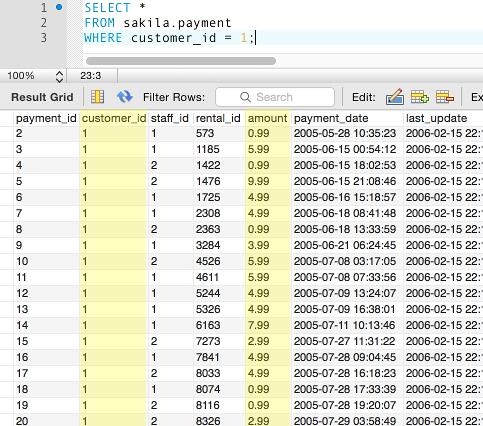The above table stores customers' payment data. Each time a customer makes a payment, a new record is added to this table with the payment details.

In particular, look at the `customer_id` and the `payment` columns. You can see that there are many records containing the same `customer_id`, each with a corresponding `amount`.

What if you wanted to add up the total of all entries in the `amount` column?

We could construct the following query:

Result: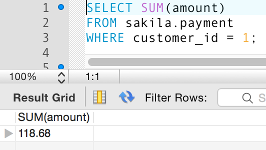This tells us that customer 1 has spent a total of \$118.68.

## All Customers

We could extend the previous example to display all customers who've made a payment. We could display one record for each user, along with the total amount that they've paid in movie rentals. Like this:

Result: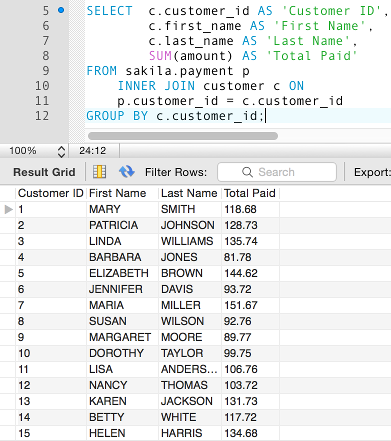In the above example, we use an `INNER JOIN` to return data from two tables (so that we can display their names along with their totals). We also provide a column heading using the `AS` clause. We use `GROUP BY` so that we can list one record for each customer, combined with their total paid. And of course, the `SUM()` function is used to provide each total.

## Add `HAVING` and `ORDER BY`

We could extend the above example even further by adding the `HAVING` and `ORDER BY` clauses.

Example:

Result: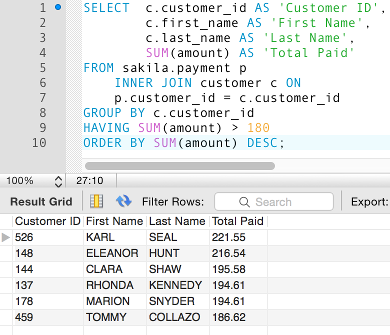In the above example, we return only those records with where the total amount exceeds 180, then order it by the amount in descending order (so that the customer who's spent the most is listed at the top).